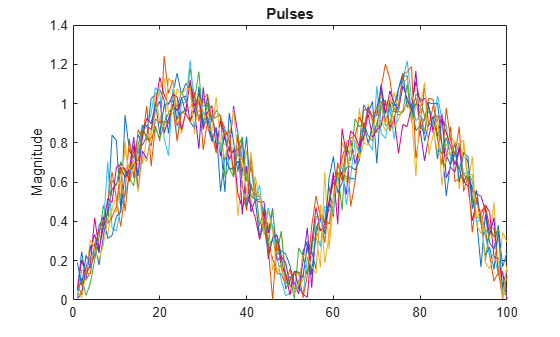# pulsint

Pulse integration

## Syntax

```Y = pulsint(X) Y = pulsint(X,METHOD) ```

## Description

`Y = pulsint(X)` performs video (noncoherent) integration of the pulses in `X` and returns the integrated output in `Y`. Each column of `X` is one pulse.

`Y = pulsint(X,METHOD)` performs pulse integration using the specified method. `METHOD` is `'coherent'` or `'noncoherent'`.

## Input Arguments

 `X` Pulse input data. Each column of `X` is one pulse. `METHOD` Pulse integration method. `METHOD` is the method used to integrate the pulses in the columns of `X`. Valid values of `METHOD` are `'coherent'` and `'noncoherent'`. The values are not case sensitive. Default: `'noncoherent'`

## Output Arguments

 `Y` Integrated pulse. `Y` is an N-by-1 column vector where N is the number of rows in the input `X`.

## Examples

collapse all

Noncoherently integrate 10 pulses of a sinusoid with added gaussian white noise.

```npulse = 10; x = repmat(sin(2*pi*(0:99)'/100),1,npulse) + 0.1*randn(100,npulse); y = pulsint(x);```

Plot a single pulse and then the integrated pulses.

```subplot(2,1,1) plot(abs(x(:,1))) ylabel('Magnitude') title('First Pulse') subplot(2,1,2) plot(abs(y)) ylabel('Magnitude') title('Integrated Pulse')```collapse all

### Coherent Integration

Let Xij denote the (i,j)-th entry of an M-by-N matrix of pulses X.

The coherent integration of the pulses in X is:

`${Y}_{i}=\sum _{j=1}^{N}{X}_{ij}$`

### Noncoherent (video) Integration

Let Xij denote the (i,j)-th entry of an M-by-N matrix of pulses X.

The noncoherent (video) integration of the pulses in X is:

`${Y}_{i}=\sqrt{\sum _{j=1}^{N}|{X}_{ij}{|}^{2}}$`

## References

 Richards, M. A. Fundamentals of Radar Signal Processing. New York: McGraw-Hill, 2005.